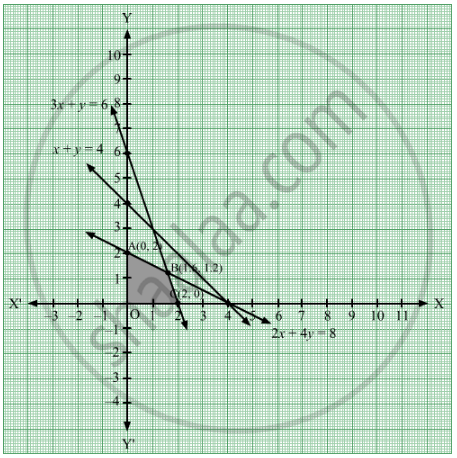# Find Graphically, the Maximum Value of Z = 2x + 5y, Subject to Constraints Given Below - Mathematics

Find graphically, the maximum value of z = 2x + 5y, subject to constraints given below :

2x + 4y  83

x + y  6

x + y  4

x  0, y 0

#### Solution

The given constraints are
2x + 4y ≤ 8   .....(1)
3x + y ≤ 6     .....(2)
x + y ≤ 4      .....(3)
x ≥ 0, y ≥ 0

We need to maximise the objective function z = 2x + 5y.

Converting the inequations into equations, we obtain the lines 2x + 4y = 8, 3x + y = 6, x + y = 4, x = 0 and y = 0.

These lines are drawn and the feasible region of the LPP is shaded.The coordinates of the corner points of the feasible region are O(0, 0), A(0, 2), B(1.6, 1.2) and C(2, 0).

The value of the objective function at these points are given in the following table.

 Points Value of the objective function z = 2x + 5y O(0, 0)A(0, 2)B(1.6, 1.2)C(2, 0) 2 × 0 + 5 × 0 = 02 × 0 + 5 × 2 = 102 × 1.6 + 5 × 1.2 = 9.22 × 2 + 5 × 0 = 4

Out of these values of z, the maximum value of z is 10 which is attained at the point (0, 2). Thus, the maximum value of z is 10.

Concept: Graphical Method of Solving Linear Programming Problems
Is there an error in this question or solution?